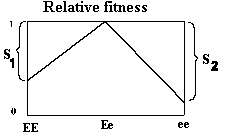### 3.3 Selection for heterozygotes

Preceding page

By selecting for heterozygotes (overdominance) the following table is obtained. Selection against the recessive (s2) and the dominant (s1) is relative in comparison to the heterozygote types.

```Table formulating selection for heterozygotes:
---------
Genotype         EE               Ee             ee       Total
Frequency        p2               2pq            q2       = 1,00
Fitness          1-s1              1            1-s2
Proportion       p2(1-s1)         2pq           q2(1-s2)   = 1-p2s1 - q2s2
after selection
---------
```

After selection the gene frequency is calculated by the gene counting method as shown in section 3.2.

```              q' = (2q2*(1-s2) + 2pq)/(2*(1-p2s1 - q2s2))
```

The frequency q' represents the genes that survive and therefore corresponds to the gene frequency in the next generation before selection. In this case, concerning overdominance, selection will not end by fixation of one of the alleles, instead an equilibrium with constant gene frequencies will occur.
The equilibrium frequency is called q (hat), and equilibrium is reached when no change occurs from one generation to the next, i.e. delta q = 0

```          delta q = q' - q = pq(ps1-qs2)/(1-p2s1 - q2s2) = 0
ps1-qs2                = 0

which solved with respect to q gives the equilibrium frequency

q(hat) = s1 / (s1 + s2) or
p(hat) = s2 / (s1 + s2)```

Delta q equals the change in the gene frequency from one generation to the next. When the gene frequency is larger than the equilibrium gene frequency q (hat) delta q becomes negative, and when gene frequency is lower delta q is positive. Therefore selection for heterozygotes is a neverending selection. Thus the population should carry a large genetic load, it is costly to maintain that type of polymorphism. Overdominance is best utilized in animal breeding by producing crossbreeds, in which all individuals can be heterozygotes.
 Figure 3.4. Fitness conditions by overdominance.Fitness by overdominance is visualized in Figure 3.4, here the two homozygote types have a fitness level which is lower than the heterozygotes, whose fitness level is 1.

Example: The classical example from human genetics of overdominance is the occurrence of the Mendelian inherited recessive sickle cell anaemia with a frequency of around 5% corresponding to q = 0.22 in malaria areas. Individuals which are heterozygotic for the sickle cell anaemia are resistant to malaria, this gives them a greater chance of survival than normal individuals. Individuals with anaemia have low chances of survival, s2=1.
What is the fitness level for the normal homozygotes in comparison to the heterozygotes?

```Equilibrium occurs at    p(hat) = s2 / (s1 + s2) = 1 - q =1 - 0.22

which gives          s1 = (s2 /(1 - q)) - s2 = 0.285```

Fitness in normal individuals in a malaria area is 1 - 0.285 = 71,5 % in comparison to the heterozygotes. The 'genetic load' of the population is p2s1 + q2s2 = 0.22, which means that 22% of a generation succumb to maintain the equilibrium, either because of  the anaemia or the malaria.

Applet for calculation of gene frequencies for different fitness combinations, click here